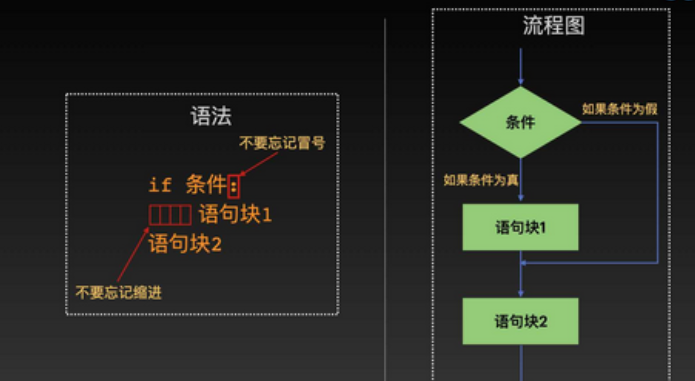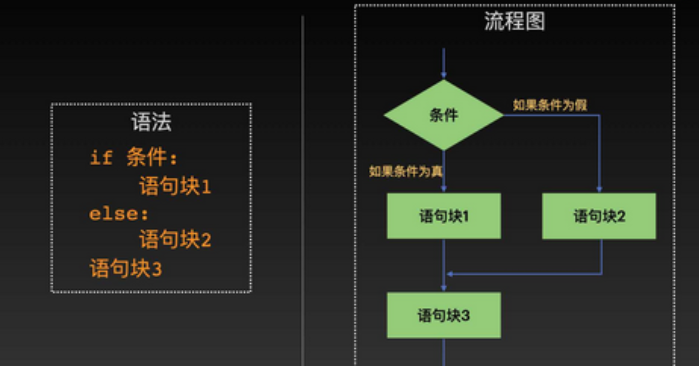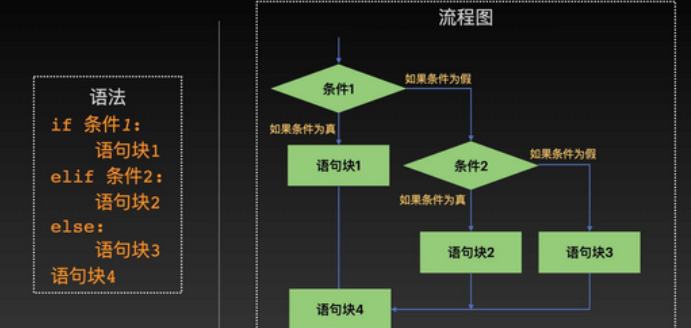# Python 语法之流程控制

• 2022 年 9 月 22 日
四川
• 本文字数：2720 字

阅读完需：约 9 分钟

# 织女的生活状态是厌烦了每天织布的枯燥生活living_conditions = '织女厌烦了每天织布的枯燥生活'if living_conditions == '织女厌烦了每天织布的枯燥生活':    print('织女下到凡间')

## 1.if 语法

#### 1.1 ifweight = 65height = 1.55BMI = float(weight) / (float(height) * float(height))if BMI >= 25:    print("BMI=", BMI)    print("织女体重过重")

#### 1.2 if... elseweight = 40height = 1.55BMI = float(weight) / (float(height) * float(height))if 18.5 <= BMI < 25:    print("BMI=", BMI)    print("织女体重正常")else:    print("织女体重过重或过轻")

#### 1.3 if...elif...elseelif 语句块可出现一次或多次。下面我们针对织女的需求，进行体重过重和过轻的进一步区分：

weight = 40height = 1.55BMI = float(weight) / (float(height) * float(height))if 18.5 <= BMI < 25:    print("BMI=", BMI)    print("织女体重正常")elif BMI < 18.5:    print("BMI=", BMI)    print("织女体重过轻")else:    print("BMI=", BMI)    print("织女体重过重")

weight = 40height = 1.55BMI = float(weight) / (float(height) * float(height))if BMI < 15:    print("BMI=", BMI)    print("织女非常严重的体重不足")elif 15 <= BMI < 16:    print("BMI=", BMI)    print("织女严重体重不足")elif 16 <= BMI < 18.5:    print("BMI=", BMI)    print("织女体重过轻")elif 18.5 <= BMI < 25:    print("BMI=", BMI)    print("织女体重正常")elif 25 <= BMI < 30:    print("BMI=", BMI)    print("织女体重过重")elif 30 <= BMI < 35:    print("BMI=", BMI)    print("织女中等肥胖")elif 35 <= BMI < 40:    print("BMI=", BMI)    print("织女严重肥胖")else:    print("BMI=", BMI)    print("织女非常严重肥胖")

#### 1.4 嵌套 if 语句

weight = 40height = 1.55BMI = float(weight) / (float(height) * float(height))if 18.5 <= BMI < 25:    print("BMI=", BMI)    print("织女体重正常")elif BMI < 18.5:    print("BMI=", BMI)    if 16 <= BMI:        print("织女体重过轻")    elif 15 <= BMI < 16:        print("织女严重体重不足")    else:        print("织女非常严重的体重不足")else:    print("BMI=", BMI)    if BMI < 30:        print("织女体重过重")    elif 30 <= BMI < 35:        print("织女中等肥胖")    elif 35 <= BMI < 40:        print("织女严重肥胖")    else:        print("织女非常严重肥胖")### 向阳逐梦

InfoQ签约作者、阿里云“乘风者计划”签约博主

## 评论